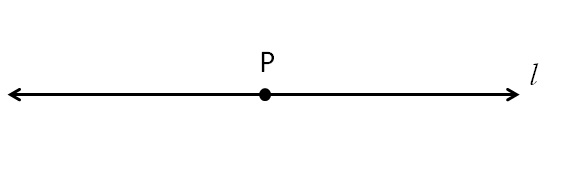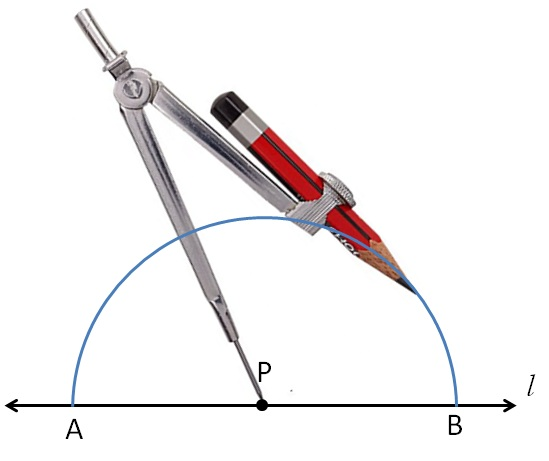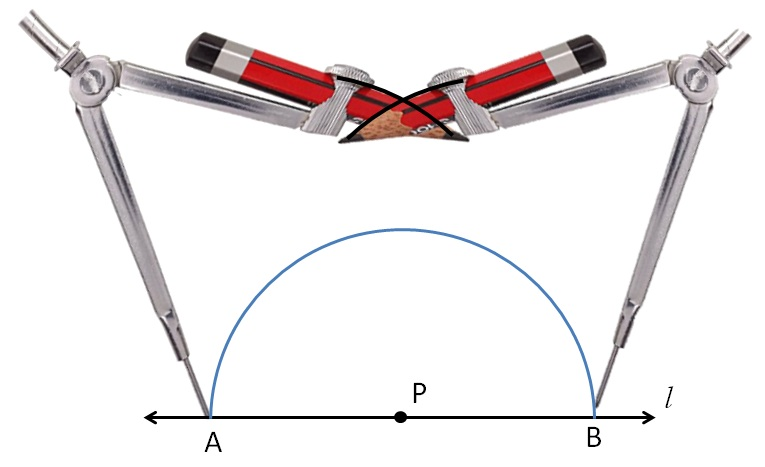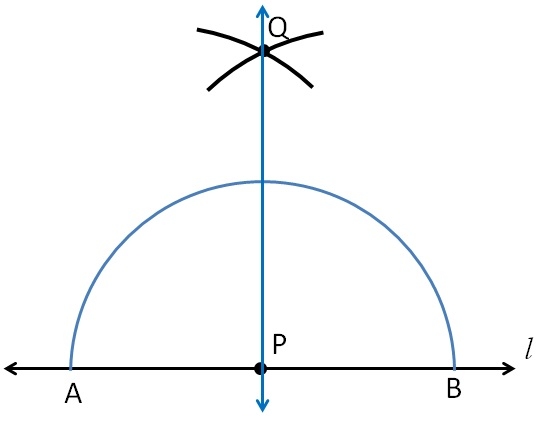Perpendicular to a line through a point on it

Chapter 14 Class 6 Practical Geometry
Concept wise

Let’s take an example

1. Given a line l
with point P marked on it1. With P as center, and any radius,
draw an arc intersecting the line at points A and B1. Now with A as center, and radius more than AP,

draw an arc.

With B as center, and same radius as before, draw an arc4.Mark the point of intersection of the two arcs as point Q Join P & Q.∴ PQ is the line perpendicular to line l

Get live Maths 1-on-1 Classs - Class 6 to 12